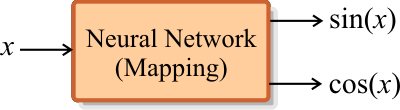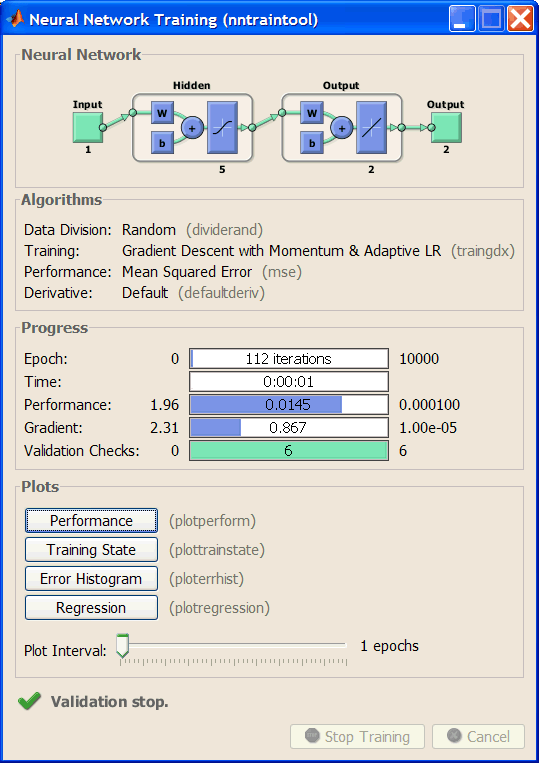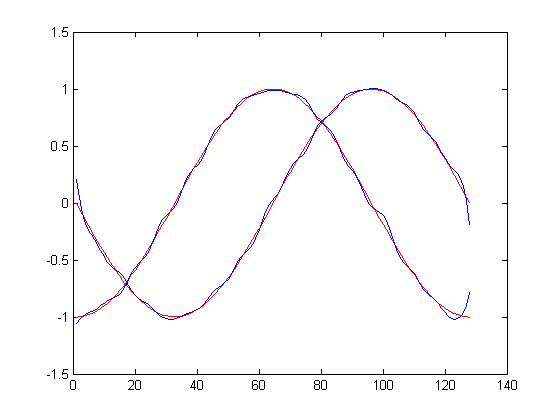# sin(x) and cos(s) using Matlab

 Problem 1 Write the BuildTrainSet.m file using Matlab to build an appropriate training set to learn the functions: sin(x) and cos(x) as shown in the figure. Build 512 training cases using uniformly distributed values for x in the range from 0 to 2π. (To keep the files organized, create the SinCosMatlab folder or all problems in this section.)BuildTrainSet.m clear; numCases = 512; delta = 2.0*pi/(numCases-1); trainSetInput = (-pi: delta : pi); % There are 2 rows and 512 columns in trainSetTarget  trainSetTarget = [sin(trainSetInput); cos(trainSetInput)];

 Problem 2 Write the BuildValidSet.m file using Matlab to build the respective validation set. Use 128 validation cases.

 BuildValidSet.m numCases = 128; delta = 2.0*pi/(numCases-1); validSetInput = (-pi: delta : pi); % There are 2 rows and 128 columns in validSetTarget  validSetTarget = [sin(validSetInput); cos(validSetInput)];

 Problem 3 Write the Trainx.m file using Matlab to design and train the ANN. Use one hidden layer and five neurons in this layer. If you do not get an acceptable mse, increase the number of neurons in the hidden layer. Train the ANN using the Conjugate Gradient method:      Number of iterations = 10000      Goal (desired mse) = 0.0001

 Trainx.m %________________________ 2010a and later net = feedforwardnet(5, 'traingdx'); net.trainParam.epochs=10000; net.trainParam.goal=0.000001; net = train(net, trainSetInput, trainSetTarget); % %________________________ Before version 2010a %ranges = [-pi, pi]; %net = newff(ranges, [5, 2], {'tansig', 'tansig'}, 'traingdx'); %net.trainParam.epochs=10000; %net.trainParam.goal=0.0001; %net = train(net, trainSetInput, trainSetTarget);Problem 4 Write the CheckTraining.m file using Matlab to check the training by computing the mean squared error for the ANN using the training set.

 CheckTraining.m %________________________ 2010a and later output = net(trainSetInput); mse(output-trainSetTarget) %________________________ Before version 2010a %output = sim(net, trainSetInput); %mse(output-trainSetTarget)

 Output > > CheckTraining ans =     0.0149

 Problem 5 Write the Validation.m file using Matlab to perform the validation of the ANN by computing the mean squared error for the ANN using the validation set.

 Validation.m %________________________ 2010a and later output = net(validSetInput); mse(output-validSetTarget) %________________________ Before version 2010a output = sim(net, validSetInput); mse(output-validSetTarget)

 Output > > Validation ans =     0.0149

 Problem 6 Write the Simulatex.m file using Matlab to simulate the ANN previously trained.

 Simulatex.m %________________________ 2010a and later Y = net(validSetInput); results = [Y; validSetTarget]; hold on; plot(validSetTarget', 'r'); plot(Y', 'b'); hold off; %________________________ Before version 2010a %Y = sim(net, validSetInput); %results = [Y; validSetTarget]; %hold on; %plot(validSetTarget', 'r'); %plot(Y', 'b'); %hold off;